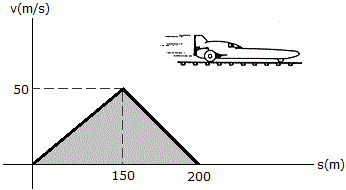Engineering Mechanics - Kinematics of Particle (KOP) - Discussion

Discussion :: Kinematics of Particle (KOP) - General Questions (Q.No.7)

7.The v-s graph for a rocket sled is shown. Determine the acceleration of the sled when s = 100 m and s = 175 m.

 [A]. a100 = 3.75 m/s2, a175 = -1.250 m/s2 [B]. a100 = 11.11 m/s2, a175 = -25.0 m/s2 [C]. a100 = 0.333 m/s2, a175 = -1.000 m/s2 [D]. a100 = 33.3 m/s2, a175 = -25 m/s2

Explanation:

No answer description available for this question.

 Rajkiran Hatheshwar said: (Dec 6, 2015) Step 1 - By similar triangle at s=100m, we will get v=(100/3)m/s. Now from s=d/t; find t=3 sec. Then from v=u+at, we will get a=11.11 m/s*s (here u=0). Step 2 - Similarly at s=175m, v=25m/s and t=1sec. So that a=-25m/s*s. Note:- in 2nd case you will not be 0, here you will be 50m/s. THANK YOU.

 Nayan said: (Feb 1, 2017) Please explain how you taking values of t and v?

 Pradeep said: (Feb 27, 2018) @Nayan it is a concept of the similar triangle: which implies 150/50=100/v. Gives v=100/3 and from s=v*t we will get t.

 Raj Shah said: (Nov 25, 2020) Let s0=0m, s1=150m, s2=200m, s3=100m, & s4=175m. a3=[(s3-s0)/(s1-s0)]*v1*[(v1-v0)/(s1-s0)]. a3=11.11m/s*s. a4=[(s4-s1)/(s2-s1)]*v1*[(v2-v1)/(s2-s1)]. a4= -25 m/s*s.